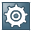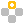Show TOC

###Rounding Rules for NutrientsIf you use nutrients as components, you can define rounding rules for quantities or recommended daily allowances (RDA) of nutrients in specification management in the PLM Web UI.

The system uses rounding rules when it determines the declared values for the component list from the calculated values.

The system uses the base unit of measure of the nutrient when rounding quantities, and rounds recommended daily allowances as percentages.

If the system cannot determine any rounding rules or any valid rounding rules for a nutrient, it rounds the values as specified in the Business Add-In (BAdI) BAdI: Data for Nutrition Label/Quantitative Component Label: Execute Rules; in the standard system, this is to two decimal places. You also use this BAdI to specify how the rounding rules are defined.

In the standard system, you define rounding rules by specifying the settings and the beginning of the intervals in which the rounding rules are applied:

• Intervals

You specify intervals that build on each other. The interval with the smallest value is the starting point from where rounding is applied. Subsequent intervals specify sections in which the rounding rule might be defined differently.

The last interval with the highest number is used for all numbers above the interval limit. The interval limit is defined as exact as four decimal places.Example

You have defined three rounding rules. You have specified the lower limit as Limit1 and Limit2. The system evaluates any calculated value to be rounded based on the following limits:

• If the value is smaller than Limit1, no rounding is applied.

• If the value is equal to or bigger than Limit1 and smaller than Limit2, the rounding defined for Limit1 is applied.

• If the value is equal to or bigger than Limit2, the rounding defined for Limit2 is applied.

End of the example.
• Settings for rounding rules

• Round to the Nearest:

The value of this field defines a stepping in which results are calculated.Example

• Round to the nearest is 5.

If the calculated value is 4, it is rounded up to 5. If the value is 7, it is rounded down to 5.

• Round to the nearest is 0.2.

If the calculated value is 8.15, it is rounded up to 8.2. If the value is 7.91, it is rounded down to 8.0.

End of the example.
• Declared Value and Declared Value Statement:

You can enter a fixed numerical value, a phrase, or a combination of both, that is used for values of this interval.Example

You have set up a rounding rule as follows:

• Interval From: 100.0001

• Declared Value: 100.00

• Declared Value Statement: More than

If the calculated value is 112.2456, then the returning value is 100.00 and the value statement is More than.

End of the example.
• Do Not Display Item:

If this field is selected, the result will be that the Display checkbox is not selected.